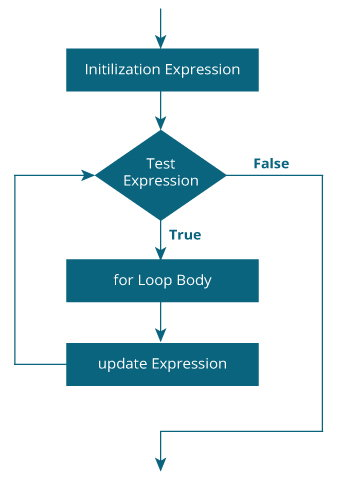# C for Loop

#### In this tutorial, you will learn to create for loop in C programming with the help of examples.

In programming, a loop is used to repeat a block of code until the specified condition is met.

C programming has three types of loops:

1. for loop
2. while loop
3. do...while loop

We will learn about `for` loop in this tutorial. In the next tutorial, we will learn about `while` and `do...while` loop.

## for Loop

The syntax of the `for` loop is:

``````for (initializationStatement; testExpression; updateStatement)
{
// statements inside the body of loop
}``````

### How for loop works?

• The initialization statement is executed only once.
• Then, the test expression is evaluated. If the test expression is evaluated to false, the `for` loop is terminated.
• However, if the test expression is evaluated to true, statements inside the body of `for` loop are executed, and the update expression is updated.
• Again the test expression is evaluated.

This process goes on until the test expression is false. When the test expression is false, the loop terminates.

To learn more about test expression (when the test expression is evaluated to true and false), check out relational and logical operators.

### for loop Flowchart### Example 1: for loop

``````// Print numbers from 1 to 10
#include <stdio.h>

int main() {
int i;

for (i = 1; i < 11; ++i)
{
printf("%d ", i);
}
return 0;
}
``````

Output

`1 2 3 4 5 6 7 8 9 10`
1. i is initialized to 1.
2. The test expression `i < 11` is evaluated. Since 1 less than 11 is true, the body of `for` loop is executed. This will print the 1 (value of i) on the screen.
3. The update statement `++i` is executed. Now, the value of i will be 2. Again, the test expression is evaluated to true, and the body of for loop is executed. This will print 2 (value of i) on the screen.
4. Again, the update statement `++i` is executed and the test expression `i < 11` is evaluated. This process goes on until i becomes 11.
5. When i becomes 11, i < 11 will be false, and the `for` loop terminates.

### Example 2: for loop

``````// Program to calculate the sum of first n natural numbers
// Positive integers 1,2,3...n are known as natural numbers

#include <stdio.h>
int main()
{
int num, count, sum = 0;

printf("Enter a positive integer: ");
scanf("%d", &num);

// for loop terminates when num is less than count
for(count = 1; count <= num; ++count)
{
sum += count;
}

printf("Sum = %d", sum);

return 0;
}``````

Output

```Enter a positive integer: 10
Sum = 55```

The value entered by the user is stored in the variable num. Suppose, the user entered 10.

The count is initialized to 1 and the test expression is evaluated. Since the test expression `count<=num` (1 less than or equal to 10) is true, the body of `for` loop is executed and the value of sum will equal to 1.

Then, the update statement `++count` is executed and the count will equal to 2. Again, the test expression is evaluated. Since 2 is also less than 10, the test expression is evaluated to true and the body of `for` loop is executed. Now, the sum will equal 3.

This process goes on and the sum is calculated until the count reaches 11.

When the count is 11, the test expression is evaluated to 0 (false), and the loop terminates.

Then, the value of `sum` is printed on the screen.

We will learn about `while` loop and `do...while` loop in the next tutorial.